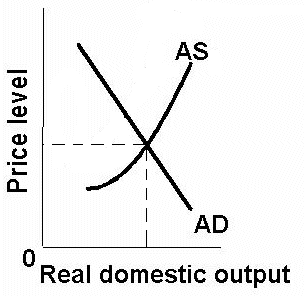1. Assume that there is an increase in government spending Which determinant? ____________ D AD or D AS?What happens to: Price Level _______________ Real GDP _______________ Inflation _______________ Unemployment _______________ Economic Growth _______________ [D GDP or D potential GDP ?] 2. Assume that there is an increase in taxes Which determinant? ____________ D AD or D AS?What happens to: Price Level _______________ Real GDP _______________ Inflation _______________ Unemployment _______________ Economic Growth _______________ [D GDP or D potential GDP ?] 3. Assume that there is reduced gov’t red tape Which determinant? ____________ D AD or D AS?What happens to: Price Level _______________ Real GDP _______________ Inflation _______________ Unemployment _______________ Economic Growth _______________ [D GDP or D potential GDP ?] 4. Assume that there is an increase in business investment Which determinant? ____________ D AD or D AS?What happens to: Price Level _______________ Real GDP _______________ Inflation _______________ Unemployment _______________ Economic Growth _______________ [D GDP or D potential GDP ?] 5. Assume that there is an increase in oil prices due to war Which determinant? ____________ D AD or D AS?What happens to: Price Level _______________ Real GDP _______________ Inflation _______________ Unemployment _______________ Economic Growth _______________ [D GDP or D potential GDP ?] 6. Assume that the Fed decreases the money supply Which determinant? ____________ D AD or D AS?What happens to: Price Level _______________ Real GDP _______________ Inflation _______________ Unemployment _______________ Economic Growth _______________ [D GDP or D potential GDP ?] 7. Assume that there is new technology that reduces electricity costs Which determinant? ____________ D AD or D AS?What happens to: Price Level _______________ Real GDP _______________ Inflation _______________ Unemployment _______________ Economic Growth _______________ [D GDP or D potential GDP ?] 8. Assume that exports increase Which determinant? ____________ D AD or D AS?What happens to: Price Level _______________ Real GDP _______________ Inflation _______________ Unemployment _______________ Economic Growth _______________ [D GDP or D potential GDP ?] 9. Assume that there is a decrease in marginal tax rates which increases labor productivity Which determinant? ____________ D AD or D AS?What happens to: Price Level _______________ Real GDP _______________ Inflation _______________ Unemployment _______________ Economic Growth _______________ [D GDP or D potential GDP ?] 10. Assume that there is an poor harvests world wide which increases resource prices Which determinant? ____________ D AD or D AS?What happens to: Price Level _______________ Real GDP _______________ Inflation _______________ Unemployment _______________ Economic Growth _______________ [D GDP or D potential GDP ?] 11. Assume that there is a rise in the foreign exchange value of the dollar Which determinant? ____________ D AD or D AS?What happens to: Price Level _______________ Real GDP _______________ Inflation _______________ Unemployment _______________ Economic Growth _______________ [D GDP or D potential GDP ?] 12. Assume that there is an increase in labor training and education Which determinant? ____________ D AD or D AS?What happens to: Price Level _______________ Real GDP _______________ Inflation _______________ Unemployment _______________ Economic Growth _______________ [D GDP or D potential GDP ?] 13. Assume that there is an increase in consumer debt Which determinant? ____________ D AD or D AS?What happens to: Price Level _______________ Real GDP _______________ Inflation _______________ Unemployment _______________ Economic Growth _______________ [D GDP or D potential GDP ?] 14. Assume that there is a decrease in consumer confidence Which determinant? ____________ D AD or D AS?What happens to: Price Level _______________ Real GDP _______________ Inflation _______________ Unemployment _______________ Economic Growth _______________ [D GDP or D potential GDP ?] 15. Assume that there is an recessions in Europe, Japan, and Canada Which determinant? ____________ D AD or D AS?What happens to: Price Level _______________ Real GDP _______________ Inflation _______________ Unemployment _______________ Economic Growth _______________ [D GDP or D potential GDP ?] 16. Assume that there are discoveries of new oil fields Which determinant? ____________ D AD or D AS?What happens to: Price Level _______________ Real GDP _______________ Inflation _______________ Unemployment _______________ Economic Growth _______________ [D GDP or D potential GDP ?] 17. Assume that there is an increase in interest rates not caused by price level changes Which determinant? ____________ D AD or D AS?What happens to: Price Level _______________ Real GDP _______________ Inflation _______________ Unemployment _______________ Economic Growth _______________ [D GDP or D potential GDP ?] 18. Assume that there is a decrease in the amount of money in circulation Which determinant? ____________ D AD or D AS?What happens to: Price Level _______________ Real GDP _______________ Inflation _______________ Unemployment _______________ Economic Growth _______________ [D GDP or D potential GDP ?] 19. Assume that there is an international agreement to make businesses reduce pollution Which determinant? ____________ D AD or D AS?What happens to: Price Level _______________ Real GDP _______________ Inflation _______________ Unemployment _______________ Economic Growth _______________ [D GDP or D potential GDP ?] 20. Assume that there is an more competition due to fewer trade restrictions Which determinant? ____________ D AD or D AS?What happens to: Price Level _______________ Real GDP _______________ Inflation _______________ Unemployment _______________ Economic Growth _______________ [D GDP or D potential GDP ?]